# Fixed Mask Not Working as Expected

Hi all,

Is the following a “bug” which should be addressed or a “feature” which should be circumvented:

When using the fixed image mask as part of registration I expect that only points that are inside the mask will be used and that the size of the image is irrelevant to the registration as I am concerned with a sub-region of the image defined by the mask.

Apparently this is incorrect, the points used are indeed only those inside the mask, but the image size effects the registration. This is because of the sampling approach implemented in the image registration method, rejection sampling instead of sampling directly from the mask.

Below you can see two sets of images with a rectangular object of interest. The two sets are identical except that one has a larger extent than the other. The results I get when running 1000 registration runs on these images are:

``````small image errors x mean(std), errors y mean(std): 0.3870545057599005 (1.0506900516481392) 0.8770167849487113 (1.6907735104396913)
large image errors x mean(std), errors y mean(std): 8.954321203919642 (197.4478418702482) 7.28542048869902 (33.27088769758669)
``````

Also, for the larger image extent, once in a while the registration will throw an exception because no valid points are available for estimating the similarity measure.

Small image extent data:
fixed image: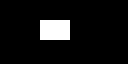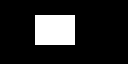moving image: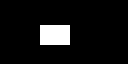Large image extent data:
fixed image: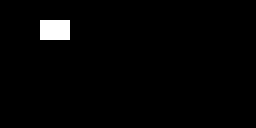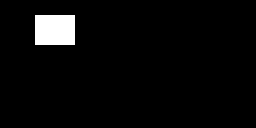moving image: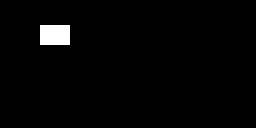In this case it is easy to circumvent the issue by cropping to the mask, but if I have a sparse mask this won’t work that well, but I can always increase the number of samples so eventually enough of them will fall inside the mask.

Would appreciate any insights into the rationale for using rejection sampling instead of sampling the points from the mask, and whether this is a “feature” or “bug”.
thanks
Ziv

p.s. This post is already a bit long so I am omitting my MWE in SimpleITK. Let me know if it will help clarify the issue and I will post the code.

3 Likes

Are you using random or other such sampling or dense “all points” sampling?

There was a discussion about this or something very similar in July. And it resulted in a patch which was merged.

Random, all points wouldn’t be sampling would it@zivy Looks like you have two things mixed together, the failing out on points has been resolved by the patch mentioned by @dzenanz

Can you re-run your tests with an updated ITK to eliminate that issue?

Unfortunately this isn’t the same issue. I’m using the simplest registration setup I could (the patch appears to be specific to mutual information which I’m not using).

The current issue is more generic/fundamental to the registration framework - it is not a problem with a specific metric it is a “feature” of the ImageRegistrationMethodv4 (see the link in my original post). The use of the mask in the larger image is expected to limit the sampling so that it isn’t across the whole image, only inside the mask. The use of rejection sampling to do this results in too few samples, so registration fails even when the user is providing enough information for it to succeed. In this example I expected the mask to be equivalent to cropping the fixed image to the ROI.

The SimpleITK code illustrating the issue is below so that you have all of the information (see which optimizer/transform/mask I use + any bugs I have) .

``````import SimpleITK as sitk
import numpy as np

alpha = 0.5

####################################
# Register the two images, starting with the identity transform. The two
# images completely overlap.
####################################
transform = sitk.TranslationTransform(2)

registration_method = sitk.ImageRegistrationMethod()

registration_method.SetMetricAsCorrelation()
registration_method.SetMetricSamplingStrategy(registration_method.RANDOM)

number_samples = 256

percentage = number_samples/ np.prod(fixed_image.GetSize())

registration_method.SetMetricSamplingPercentage(percentage)
registration_method.SetOptimizerScalesFromPhysicalShift()

registration_method.SetInitialTransform(transform, inPlace=True)

final_transform = registration_method.Execute(sitk.Cast(fixed_image, sitk.sitkFloat32),
sitk.Cast(moving_image, sitk.sitkFloat32))
return transform.GetOffset()

####################################
# Create images, fixed and moving and the fixed image mask.
# Image content is a rectangle which is shifted by dx,dy in the moving image.
####################################

rect_width = 30
rect_height = 20
rect_start_x = 40
rect_start_y = 20

npa = np.zeros((img_height, img_width))
npa[rect_start_y:rect_start_y+rect_height,rect_start_x:rect_start_x+rect_width] = 255
fixed_image = sitk.GetImageFromArray(npa)

npa = np.zeros((img_height, img_width))
npa[rect_start_y+dy:rect_start_y+rect_height+dy,rect_start_x+dx:rect_start_x+rect_width+dx] = 255
moving_image = sitk.GetImageFromArray(npa)

dx = 0
dy = 5

img_height = 64
img_width = 128
sitk.WriteImage(sitk.Cast(fixed_image,sitk.sitkUInt8), "small_fixed_image.png")
sitk.WriteImage(sitk.Cast(moving_image, sitk.sitkUInt8), "small_moving_image.png")
#sitk.Show((1.0 - alpha)*fixed_image + alpha*moving_image, "small combined image before registration")

errors_x = []
errors_y = []
for i in range(1000):
est_x, est_y = estimate_translation(fixed_image, moving_image, fixed_mask)
errors_x.append(np.abs(est_x - dx))
errors_y.append(np.abs(est_y - dy))

print('small image errors x mean(std), errors y mean(std): {0} ({1}) {2} ({3})'.format(np.mean(errors_x), np.std(errors_x), np.mean(errors_y), np.std(errors_y)))

img_height = 128
img_width = 256
sitk.WriteImage(sitk.Cast(fixed_image,sitk.sitkUInt8), "large_fixed_image.png")
sitk.WriteImage(sitk.Cast(moving_image,sitk.sitkUInt8), "large_moving_image.png")
#sitk.Show((1.0 - alpha)*fixed_image + alpha*moving_image, "large combined image before registration")

errors_x = []
errors_y = []
for i in range(1000):
est_x, est_y = estimate_translation(fixed_image, moving_image, fixed_mask)
errors_x.append(np.abs(est_x - dx))
errors_y.append(np.abs(est_y - dy))

print('large image errors x mean(std), errors y mean(std): {0} ({1}) {2} ({3})'.format(np.mean(errors_x), np.std(errors_x), np.mean(errors_y), np.std(errors_y)))

``````

So, is the issue of rejection sampling that samples are rejected and so the total number of samples reduced?

If so, should be simple enough to not increment the sample count if the point is rejected and random sample until the right number is achieved inside the mask.

@gdevenyi Yes, this is what I believe is happening and as I alluded in my original post, increasing the number of samples will improve the situation, but not in a deterministic way. This is why I was not sure if we classify the issue as “feature” or “bug”.

I was also looking for rationale for the choice of rejection based sampling vs. sampling directly from the mask. Even a “we thought about it and it was easier to implement” is reasonable, making the issue a “feature”. Otherwise it is just a random artifact which is not documented.

If this is determined to be a “feature” then we need to document it, indicating that the number of samples depends on the image size even when using a mask (larger image means you need to use a larger sample size even if you specify a small ROI, something that to me is counterintuitive).

Okay, digging into the code here:

To make it explicit now on what I think @zivy is seeing, at

The total number of samples as a percentage of the virtualdomain is computed.

One thing I’m a bit confused on still is the “++index” step is still within the check for accepting the voxel, so by my read masked registration with a larger image should result in more samples being included.

Okay, so it looks like when things are masked there’s no feedback to the sampling iterator to keep trying.

Probabilistically, large images have a lower probability of sampling inside the mask, so you get less total points.

It looks like if a point is not accepted the iterator needs to be decremented so that it keeps trying until enough points are sampled.

``````else {
--ItR;
}
``````

After

Would force the iterator to keep trying to sample enough points.

My only other concern is that maybe if the registration is masked than the number of points should be a percentage of the mask rather than of the whole image.

Just to point out an issue with decrementing the iterator whenever a point is rejected by the mask:

You can get stuck there for a while as the process is not deterministic and you may generate a lot of points outside the mask till you get one inside. I’m pretty sure we will break the registration, make it unusable, when using a small mask inside a large image (a pseudo-infinite loop?).

With the current rejection sampling implementation we don’t want to limit the number of samples (percentage) based on the mask size as that decreases your chances of getting enough points in the masked region [max_points = 1.0image_size > 1.0mask_size].

Agree, decrementing the iterator could indeed end in a pseudo infinite loop.

Similarly, decreasing the needed points without sampling from within the mask would indeed be a bad ideaLooks like the best choice for the Random sampling case is to sample from within the mask, but I’m at my limits for ITK on how to do this, something about this line needs to change if a mask is specified:

Hi there,

I would like to follow up on this thread and see of there have been any updates? I’ve recently encountered this issue while trying to set the fixed image region prior to registration to help force the similarity metric to consider more relevant voxels. It seemed that setting the fixed image region had no affect on the outcome. If this issue persists, that may explain what I am seeing.

I want to see which voxels are actually sampled that contribute to the similarity metric calculation to verify that the fixed image region was applied correctly and to determine the number of voxels which are contributing. Are the methods available for doing this?

I am currently using v3 methods, e.g. ImageRegistrationMethod and MattesMutualInformationImageToImageMetric.

Thank you,
Spencer

Hello @spencermanwell,

I don’t think anything has changed since this discussion. A workaround that may work for you is to crop the fixed image to the bounding box of your region of interest.

Note that this approach may not always help (increase the percentage of valid voxels for registration in the image) or be applicable . For example if the object in the image is annulus shaped and is already tightly bounded by the original image.

My solution from this discussion was to avoid random sampling, and stick to grid-based, which don’t trigger this code path and the problems discussed.